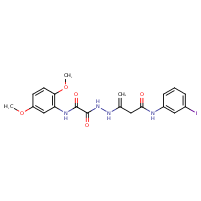Structure InformationCompound Identification

SMILES

COC1=CC(NC(=O)C(=O)NNC(=C)CC(=O)NC2=CC(I)=CC=C2)=C(OC)C=C1

InChIKey

InChIKey=VHSSYCGOAPGEKZ-UHFFFAOYSA-N

Formula

C20H21IN4O5

Mass

524.315